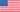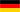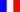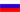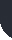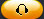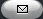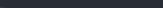Task Management Software for Team Collaboration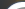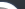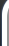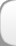HomeDownloadSupportPurchase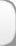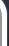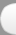Installation GuideStartUp GuideOnline Help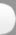# Direct Labor Efficiency Variance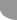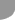Home  » Products  » VIP Task Manager Pro  » Tutorials  » Customization Guide  » Direct Labor Efficiency Variance

Direct Labor Efficiency Variance

Direct Labor Efficiency Variance (also known as Direct Labor Usage Variance or Direct Labor and Direct Labor Quantity Variance) shows the difference between the number of actual hours worked allocated per unit and the number of budgeted or standard hours allocated per unit multiplied by Standard Labor Rate. The formula to calculate Direct Labor Efficiency Variance is as follows:

Direct Labor Efficiency Variance = (Direct Labor Hours  Standard labor hours) * Standard Labor Rate

Direct Labor Hours are actual hours worked by employees. Direct Labor Hours are also used to calculate Labor Rate Variance

Standard Labor hours are estimated labor hours to produce required number of units.

If Direct Labor Hours are greater than Standard labor hours, the variance is unfavorable. If Direct Labor Hours are less than Standard labor hours, the variance is considered favorable. In other words, using the formula above, if the value is negative, Direct Labor Efficiency Variance is favorable, if the value is positive, Direct Labor Efficiency Variance is unfavorable.

For example, if a company with Standard Labor Rate \$21 USD budgeted 4000 Standard labor hours to produce 2000 units, Direct Labor Cost would be \$84,000 USD (4000 Standard labor hours * 21 USD). If the actual number of hours to produce the same number of units was reported as 3600 labor hours, Direct Labor Cost would be \$75 600 USD (3600 labor hours * \$21 USD). The hour variance was 400 labor hours (4000  3600). According to the formula Direct Labor Efficiency Variance should be calculated as follows:

Direct Labor Efficiency Variance = (Direct Labor Hours  Standard labor hours) * Standard Labor Rate = (3600 4000) * \$21 USD = -8400

The negative value would speak about favorable Direct Labor Efficiency Variance.

The sum of Direct Labor Efficiency Variance and Labor Rate Variance help you measure the total direct labor variance.

• Add custom field Direct Labor Hours (used to calculate Actual Labor Cost) with Code cf_direct_labor_hours
•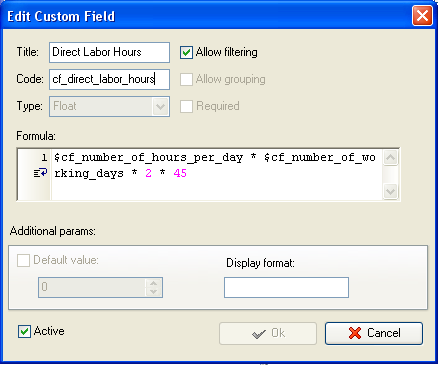• Add custom field Standard labor hours with Code cf_standard_labor_hours
•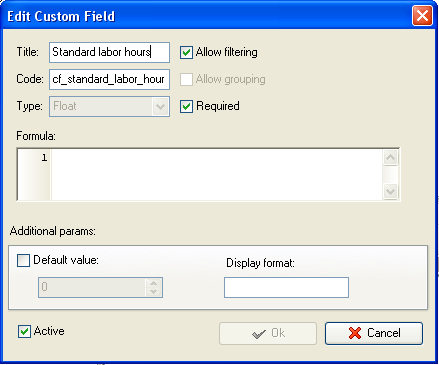• Add custom field Standard Labor Rate with Code cf_standard_labor_rate and the formula:
• \$cf_average_wage_rate + \$cf_average_employment_taxes + \$cf_average_fringe_benefits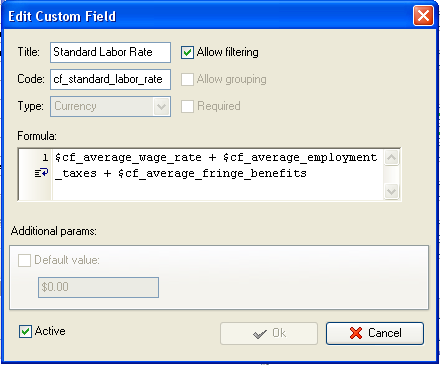• Add custom field Direct Labor Efficiency Variance  with formula:
• (\$cf_direct_labor_hours \$cf_standard_labor_hours) * \$cf_standard_labor_rate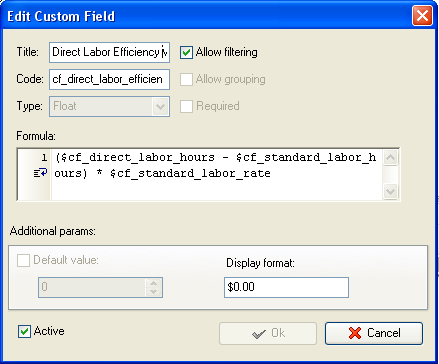• On Task Tree select task group for which you need to display Direct Labor Efficiency Variance and select Custom fields options
•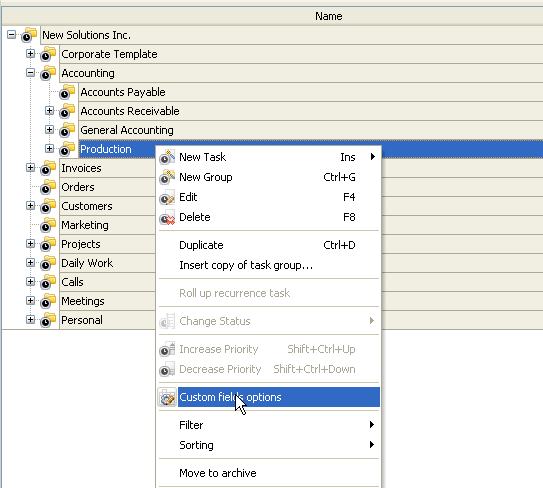• Set the following custom fields settings for the custom fields Standard Labor Hours:
• In Settings for select This task group
• select Enable
• select Edit
•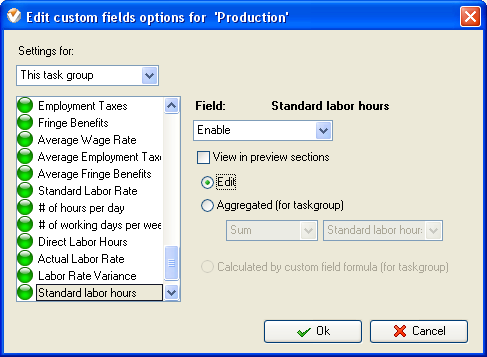• To fill in the custom fields at the task group right click on task group and select Edit
•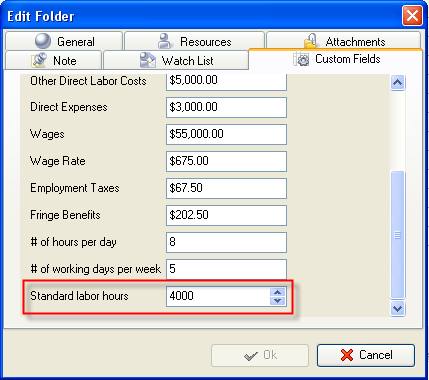• Set the following custom fields settings for the custom fields Direct labor Hours, Standard Labor Rate and Direct Labor Efficiency Variance:
• In Settings for select This task group
• select Enable
• select Calculated by custom field formula (for task group)
•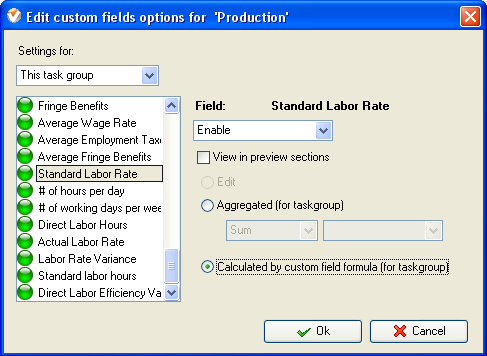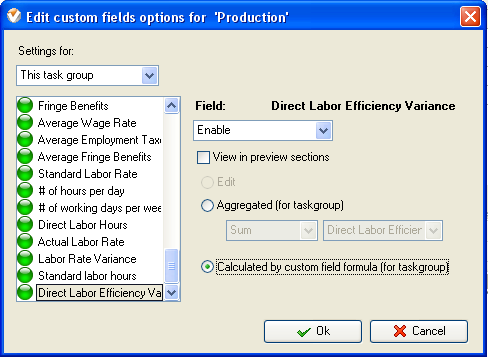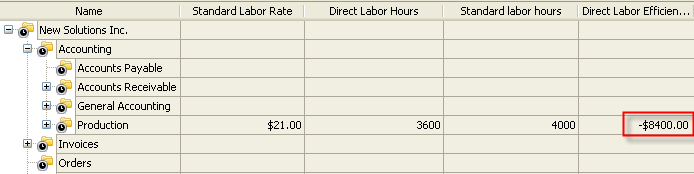Direct Labor Efficiency Variance on Task Tree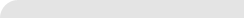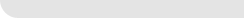» VIP Task Manager Pro VIP Task Manager Std Testimonials Turnkey Solution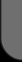ProductsSolutionsDownloadSupportPurchaseContactsForumVideoMy Account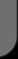Copyright © 2004 - 2023 Task Management Software